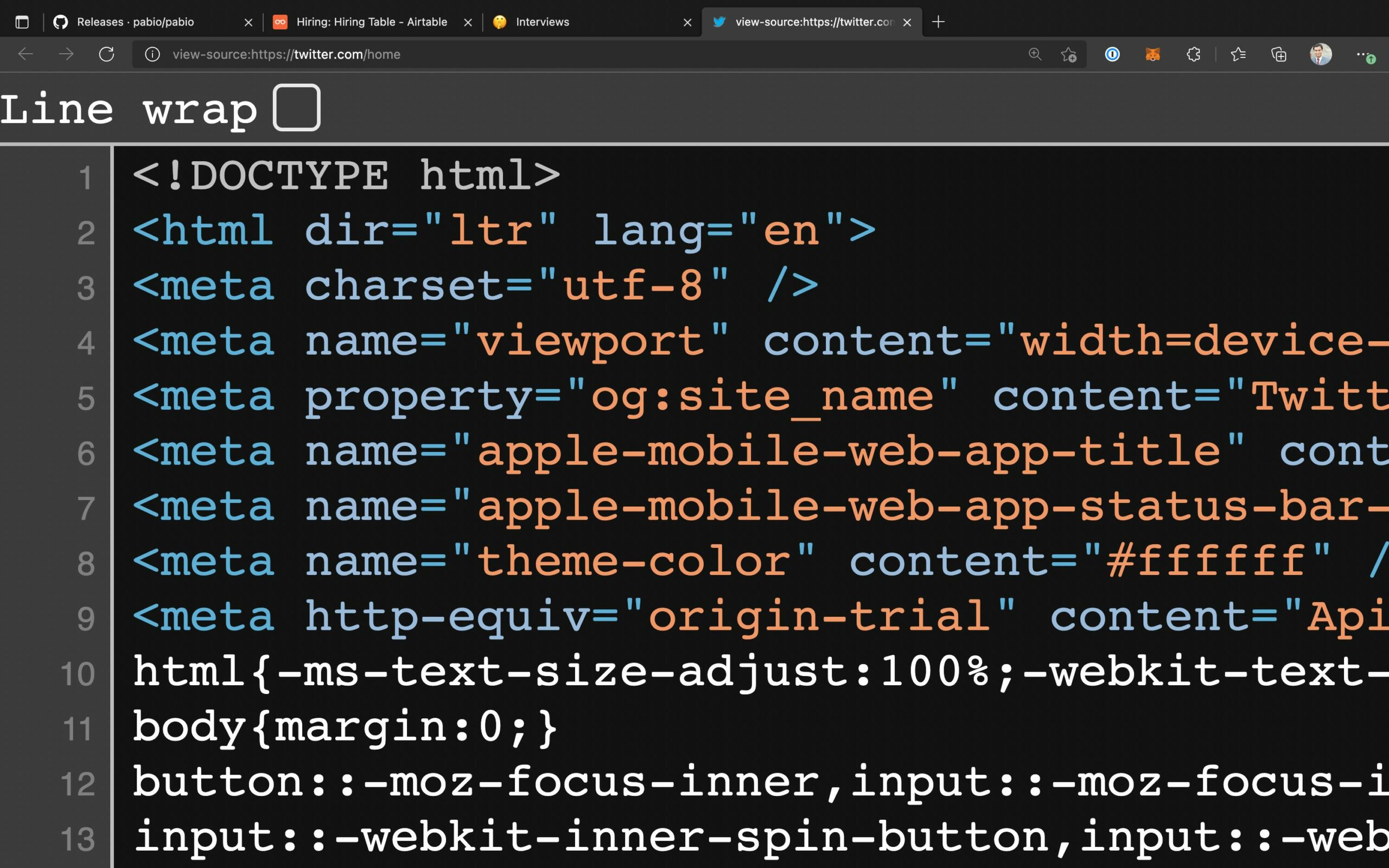Read Time:3 Minute, 43 Second

In this article, we shall take a look at generating random numbers in JavaScript with practical examples. First, we will explore the definition of Math.random() and use examples to elaborate its applications on our usual daily tasks.

## Generating random numbers in JavaScript

`JavaScript Math.random()`

The Math.random() method is responsible for generating a random number ranging from 0 (inclusive) to 1 (exclusive).

```randomNumber =Math.random()
console.log(randomNumber)```

We’ve declared a variable randomNumber and given it a random value between 0 and 1 that’s bigger than or equal to 0. Note that because Math.random() generates a random number, you can receive a different result in the above function. Using the formula, we can determine the random value between any two numbers using this value in the range (0,1).

`Math.random() * (highestNumber - lowestNumber) + lowestNumber`

### Example No. 2

Return a number between 1 and 10 at random:

```exampleVal =Math.floor((Math.random() * 10) + 1);
console.log(exampleVal)```

The example above will display a random floating-point number between 1 and 10 larger than one but less than 10. All of the examples above result in floating-point random numbers

### Example No. 3

Return a number between 1 and 100 at random:

```randomNumber = Math.floor((Math.random() * 100) + 1);
console.log(randomNumber);```

Example number 3 above also displays a random floating-point number. The latter is a value between 1 and 100.

### Use Math.floor() to generate a random integer number

Math.floor() reduces the value to the nearest integer value and returns the result.

As an example,

```Math.floor(12.389); // 12
Math.floor(12.9); // 12```

Similarly, you may use the following formula to obtain the random integer between min (inclusive) and max (inclusive):

`Math.floor(Math.random() * (max - min + 1)) + min`

### Example 4: Integer Value Between Two Numbers (Inclusive)

```// user input
const minVal = parseInt(prompt("Enter a min value: "));
const maxVal = parseInt(prompt("Enter a max value: ")); // random number generation
const a = Math.floor(Math.random() * (maxVal - minVal + 1)) + minVal; // shows a random number
console.log(Random value between \${minVal} and \${maxVal} is \${a});
```

### Obtaining a random integer between two values that is both inclusive and exclusive

While the getRandomInt() function is inclusive at its most basic level, it is exclusive at its most advanced level. What if the outcomes must be inclusive at both the minimum and maximum levels? That’s what the getRandomIntInclusive() method does.

```function getRandomNumberIntInclusive(min, max) {
minVal = Math.ceil(min);
maxVal = Math.floor(max);
return Math.floor(Math.random() * (maxVal - minVal + 1) + minVal); //The maximum is inclusive and the minimum is inclusive
}```

### Browser support

Math.random() is a feature of ECMAScript1 (ES1). In addition, all browsers fully support ES1 (JavaScript 1997):

Syntax

`Math.random()`

Parameters

Return Value
A number ranges from 0 to 1 but does not include 1.

### Random Number Between Two Values in JavaScript

We can’t use Math.random() to generate a number between two values because it doesn’t accept any arguments. We can, however, develop a custom method to generate a random integer between two values. For a more advanced level, let’s imagine making an addition game that should generate two numbers between 500 and 520.

Here’s an example of a function that may be used to generate those figures:

```const generateRandomNumbers = (minVal, maxVal) => {
return Math.floor(Math.random() * (maxVal - minVal) + minVal);
}; console.log(generateRandomNumbers(500, 520));```

As you can see, our application creates random numbers between 500 and 520. Let’s look at our program and see how it works. The first line declares the function “generateRandomNumbers().”

The lowest and maximum values of the range of numbers within which our random number should be created are passed to our function as arguments. In addition, we use the Math.random() method to generate a random number on the following line, using our “max” and “min” variables to guarantee that the number we generate is within our range.

Then, on the last line, we call generateRandomNumbers() and send the values 500 and 520. As a result, when we execute our application, we get a random number between 500 and 520.Previous post Explain the First 10 Lines of Twitter’s Source Code to MeNext post My white whale: A use case for will-change